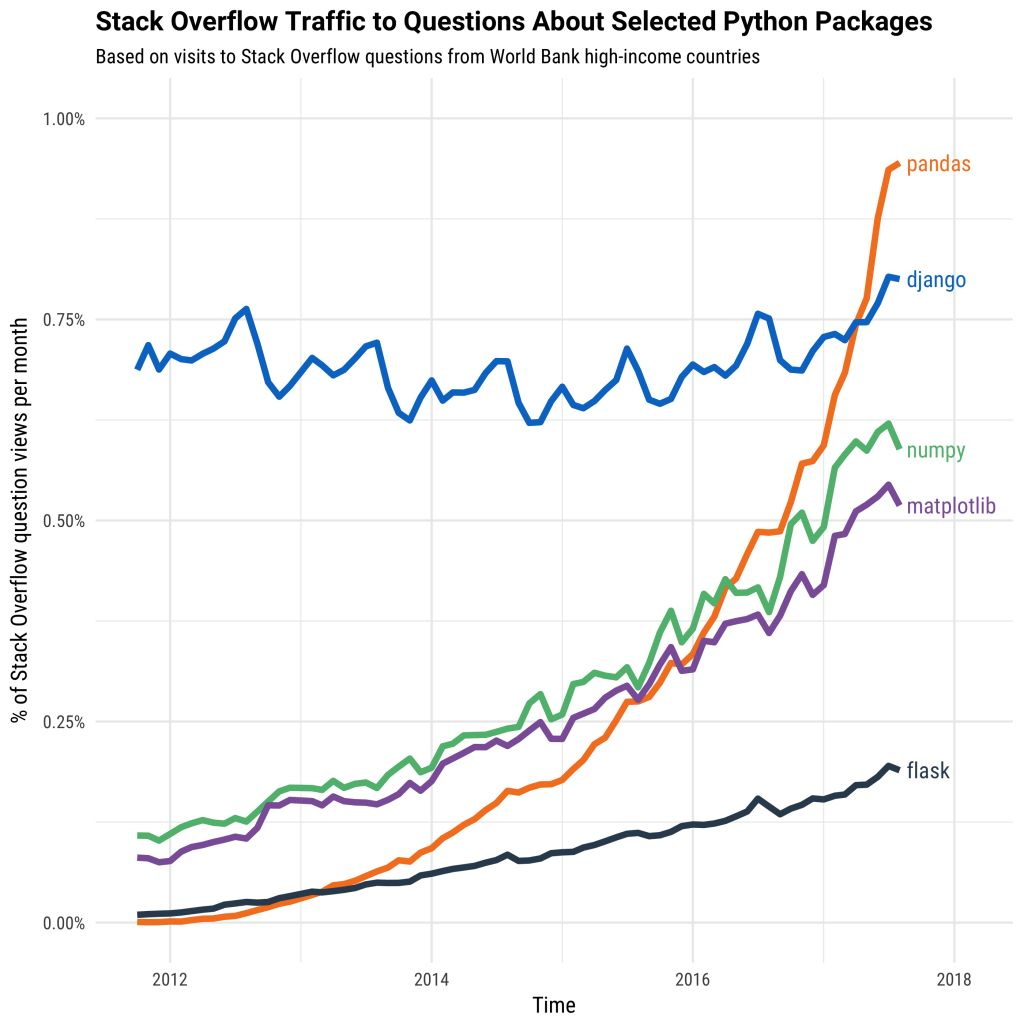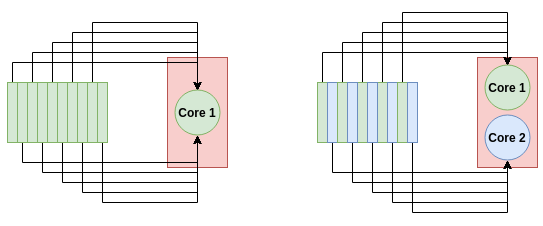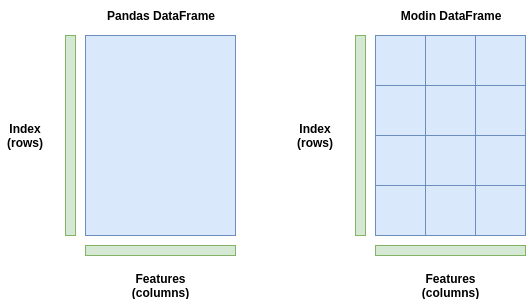# 一行代码将Pandas加速4倍Pandas是处理 Python 数据的首选库。它易于使用，并且在处理不同类型和大小的数据时非常灵活。它有大量的函数，使得操纵数据变得轻而易举。Modin是一个新的库，通过在系统所有可用的 CPU 核上自动分配计算来加速 pandas。有了它，对于任何尺寸的 pandas 数据数据集，Modin 声称能够以 CPU 内核的数量得到近乎线性的加速。

01
Modin 如何用 Pandas 并行计算panda的DataFrame(左)存储为一个块，只发送到一个CPU核。Modin的DataFrame(右)跨行和列进行分区，每个分区可以发送到不同的CPU核上，直到用光系统中的所有CPU核。

02
Modin 速度基准测试

``pip install modin[ray]``

``### Read in the data with Pandasimport pandas as pds = time.time()df = pd.read_csv("esea_master_dmg_demos.part1.csv")e = time.time()print("Pandas Loading Time = {}".format(e-s))### Read in the data with Modinimport modin.pandas as pds = time.time()df = pd.read_csv("esea_master_dmg_demos.part1.csv")e = time.time()print("Modin Loading Time = {}".format(e-s))``

``import pandas as pddf = pd.read_csv("esea_master_dmg_demos.part1.csv")s = time.time()df = pd.concat([df for _ in range(5)])e = time.time()print("Pandas Concat Time = {}".format(e-s))import modin.pandas as pddf = pd.read_csv("esea_master_dmg_demos.part1.csv")s = time.time()df = pd.concat([df for _ in range(5)])e = time.time()print("Modin Concat Time = {}".format(e-s))``

``import pandas as pddf = pd.read_csv("esea_master_dmg_demos.part1.csv")s = time.time()df = df.fillna(value=0)e = time.time()print("Pandas Concat Time = {}".format(e-s))import modin.pandas as pddf = pd.read_csv("esea_master_dmg_demos.part1.csv")s = time.time()df = df.fillna(value=0)e = time.time()print("Modin Concat Time = {}".format(e-s))``

### 警告！

Modin 总是这么快吗？

OperationPandas TimeModin TimeSpeedup
pd.concat([df for _ in range(5)])3.560.04186.83
df.groupby(by='wp_type')0.000290.0590.0049
df.fillna(value=0)1.80.218.57
df.dropna()1.241.710.73
df.count()1.090.04623.70
df.drop_duplicates()7.6813.380.57
df.describe()1.304.690.28
df['seconds'].max()0.0150.260.058
df.isnull()0.9980.01283.17
03

Modin 仍然是一个相当新的库，并在不断地发展和扩大。因此，并不是所有的 pandas 功能都被完全加速了。如果你在 Modin 中尝试使用一个还没有被加速的函数，它将默认为 panda，因此不会有任何代码错误或错误。

``import rayray.init(num_cpus=4)import modin.pandas as pd``

``export MODIN_OUT_OF_CORE=true``
04E-mail  :   contact@xinshucredit.com